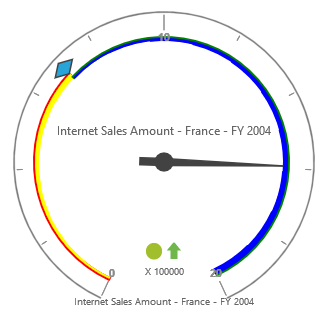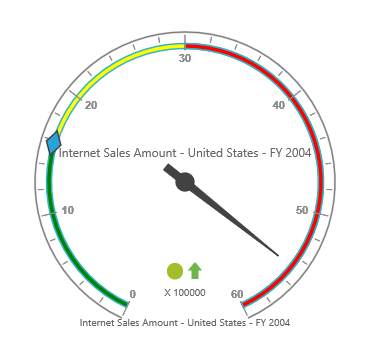# Ranges

24 May 20193 minutes to read

The `range-collection` can be directly added to the scales option within the pivot gauge control.

• CSHTML
• ``````<ej-pivot-gauge id="PivotGauge1">
<e-scales>
<e-circular-scales show-ranges="true">
<e-circular-range-collections>
<e-circular-ranges distance-from-scale="10"></e-circular-ranges>
</e-circular-range-collections>
</e-circular-scales>
</e-scales>
</ej-pivot-gauge>``````

## Appearance customization

The appearance of the range can be customized using the following properties:

• start-value: Defines the start position of the range.
• end-value: Defines the end position of the range.
• start-width: Sets the width at starting position of the range.
• end-width: Sets the width at ending position of the range.
• background-color: Sets the background color of the range.
• border: Sets the height and width of the border of the range.
• placement: Sets the position of the range.
• distance-from-scale: Sets the distance between the range and scale.

Positioning the range can be set through `placement` or `distance-from-scale` properties.

NOTE

By default, the placement takes the value near, whereas other enumeration values available are far and center.

• CSHTML
• ``````<ej-pivot-gauge id="PivotGauge1">
<e-scales>
<e-circular-scales show-ranges="true">
<e-circular-range-collections>
<e-circular-ranges start-value="20" end-value="50" start-width="2" end-width="6" background-color="yellow" distance-from-scale="20">
<e-border color="red" width="2"></e-border>
</e-circular-ranges>
<e-circular-ranges start-value="50" end-value="100" start-width="2" end-width="7" background-color="blue" placement="Near">
<e-border color="green" width="2"></e-border>
</e-circular-ranges>
</e-circular-range-collections>
</e-circular-scales>
</e-scales>
</ej-pivot-gauge>``````NOTE

When you set both the position properties - “distance-from-scale” and “placement” for a range, preference is given to the value that is set in the “distance-from-scale” property.

## Multiple ranges

Multiple ranges can be added to `range-collection` to the scales option within the pivot gauge control.

• CSHTML
• ``````<ej-pivot-gauge id="PivotGauge1">
<e-scales>
<e-circular-scales show-ranges="true">
<e-circular-range-collections>
<e-circular-ranges start-value="0" end-value="10" background-color="green" distance-from-scale="-5"></e-circular-ranges>
<e-circular-ranges start-value="10" end-value="30" background-color="yellow" distance-from-scale="-5"></e-circular-ranges>
<e-circular-ranges start-value="30" end-value="50" background-color="red" distance-from-scale="-5"></e-circular-ranges>
</e-circular-range-collections>
</e-circular-scales>
</e-scales>
</ej-pivot-gauge>``````